#`作者：Samuele Mazzanti翻译：欧阳锦校对：赵茹萱本文约3900字，建议阅读10分钟本文通过实验验证了一个通用模型优于多个专用模型的有效性的结论。`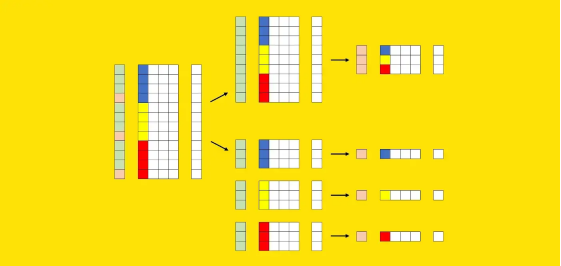• 将所有数据提供给一个模型，也就是一个通用模型（general model）；

• 为每个细分市场构建一个模型（在前面的示例中，品牌和国家/地区的组合），也就是许多专业模型（specialized models）。

# 通用模型与专用模型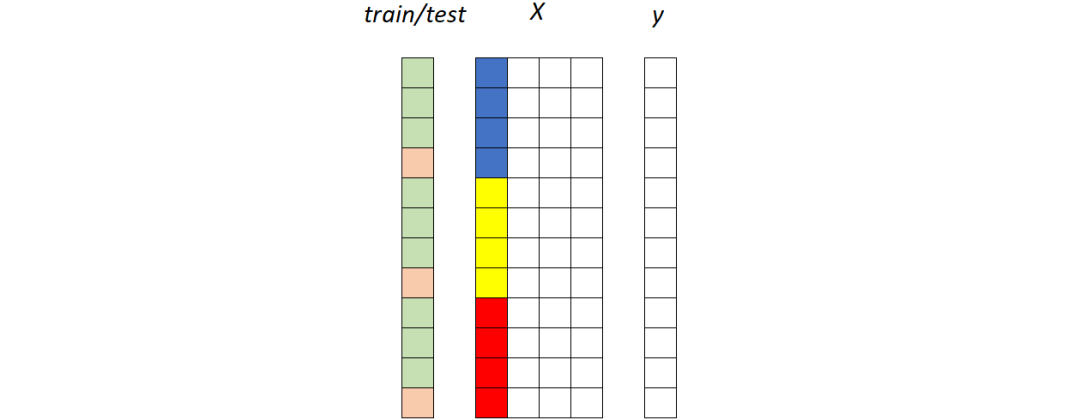## 第一种策略：通用模型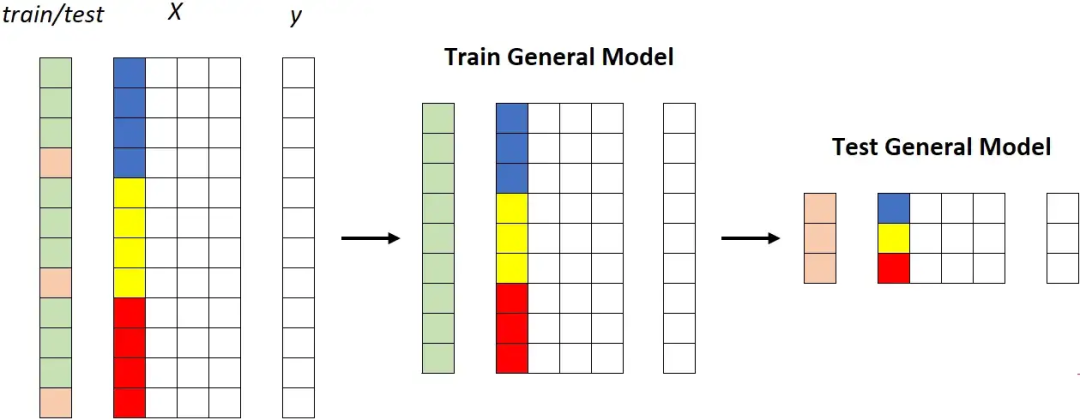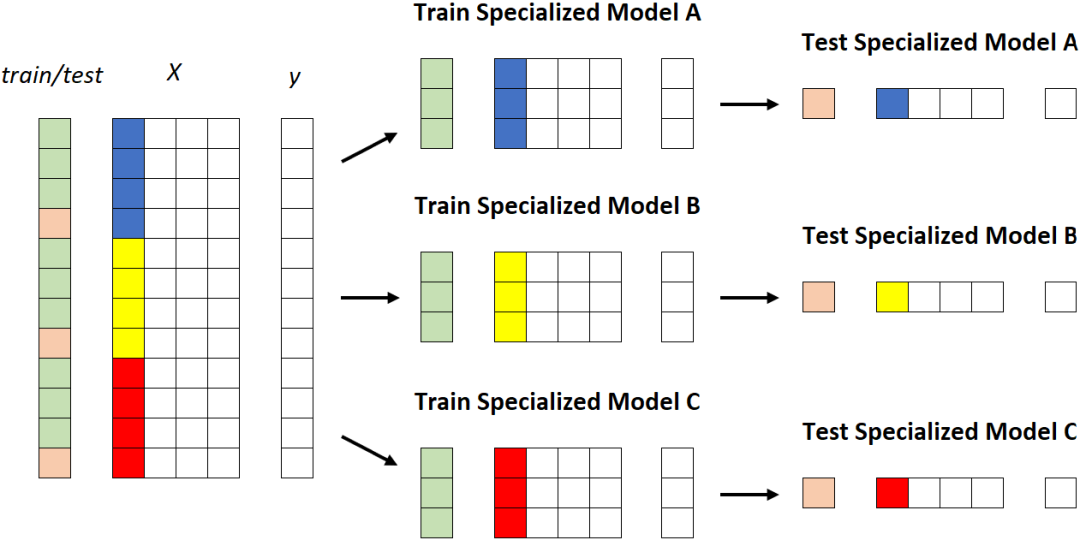专用模型。每个段被馈送到不同的模型。[作者图片]

• 更高的维护工作量；

• 更高的系统复杂度；

• 更高的（累积的）培训时间；

• 更高的计算成本：

• 更高的存储成本。

# 对通用模型的偏见

• 汽车类型（经典或现代）；

• 汽车时代；

• 车价。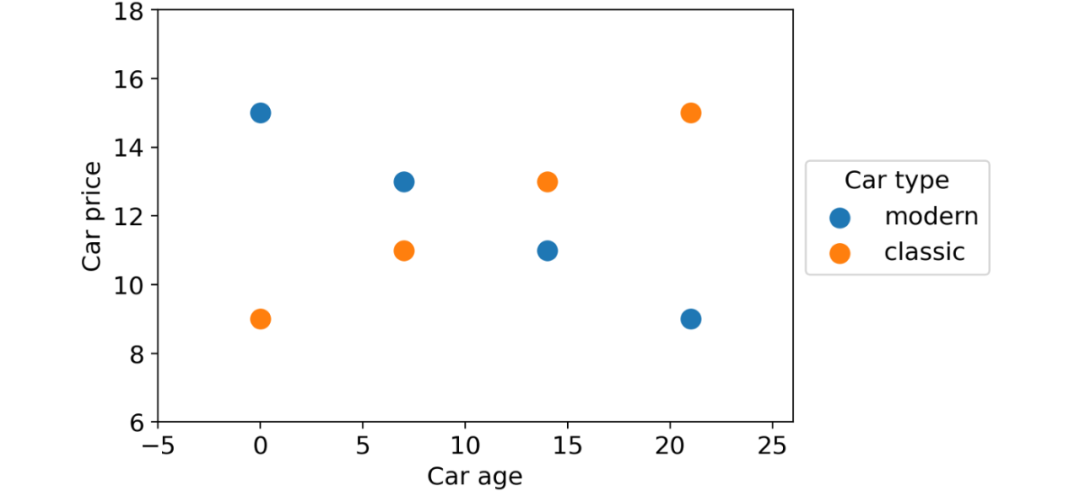``linear_regression = LinearRegression().fit(df[[ "car_type_classic" , "car_age" ]], df[ "car_price" ])``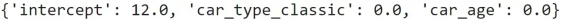``decision_tree = DecisionTreeRegressor(max_depth= 2 ).fit(df[``[ "car_type_classic" , "car_age" ]], df[ "car_price" ])``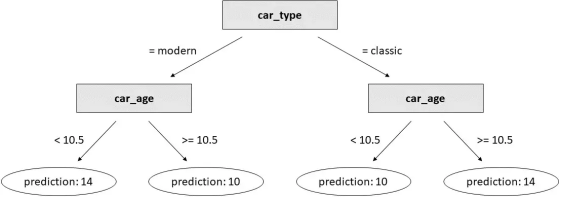# 实验细节

• 训练一个通用模型；

• 训练许多个专用模型。

1. 获取数据集；

2. 根据一列的值选择数据集的一部分；

3. 将数据集拆分为训练数据集和测试数据集；

4. 在整个训练数据集上训练通用模型；

5. 在属于该段的训练数据集部分上训练专用模型；

6. 比较通用模型和专用模型在属于该段的测试数据集部分上的性能。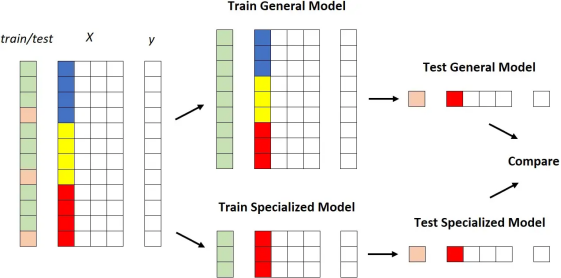X 中的彩色列是我们用来对数据集进行分层的列。[作者图片]

• 对于不同的数据集；

• 使用不同的列来分割数据集本身；

• 使用同一列的不同值来定义段。

``for each dataset:``  train general model on the training set``  for each column of the dataset:``    for each value of the column:``      train specialized model on the portion of the training set for which column = value``      compare performance of general model vs. specialized model``

``for dataset_name in tqdm(dataset_names):``  ``  # get data``  X, y, num_features, cat_features, n_classes = get_dataset(dataset_name)``  # split index in training and test set, then train general model on the training set``  ix_train, ix_test = train_test_split(X.index, test_size=.25, stratify=y)``  model_general = CatBoostClassifier().fit(X=X.loc[ix_train,:], y=y.loc[ix_train], cat_features=cat_features, silent=True)``  pred_general = pd.DataFrame(model_general.predict_proba(X.loc[ix_test, :]), index=ix_test, columns=model_general.classes_)``  # create a dataframe where all the columns are categorical: ``  # numerical columns with more than 5 unique values are binnized``  X_cat = X.copy()``  X_cat.loc[:, num_features] = X_cat.loc[:, num_features].fillna(X_cat.loc[:, num_features].median()).apply(lambda col: col if col.nunique() <= 5 else binnize(col))``  ``  # get a list of columns that are not (statistically) independent ``  # from y according to chi 2 independence test``  candidate_columns = get_dependent_columns(X_cat, y)``  ``  for segmentation_column in candidate_columns:``  ``    # get a list of candidate values such that each candidate:``    # - has at least 100 examples in the test set``    # - is not more common than 50%``    vc_test = X_cat.loc[ix_test, segmentation_column].value_counts()``    nu_train = y.loc[ix_train].groupby(X_cat.loc[ix_train, segmentation_column]).nunique()``    nu_test = y.loc[ix_test].groupby(X_cat.loc[ix_test, segmentation_column]).nunique()``    candidate_values = vc_test[(vc_test>=100) & (vc_test/len(ix_test)<.5) & (nu_train==n_classes) & (nu_test==n_classes)].index.to_list()``    ``    for value in candidate_values:``      ``      # split index in training and test set, then train specialized model ``      # on the portion of the training set that belongs to the segment``      ix_value = X_cat.loc[X_cat.loc[:, segmentation_column] == value, segmentation_column].index    ``      ix_train_specialized = list(set(ix_value).intersection(ix_train))``      ix_test_specialized = list(set(ix_value).intersection(ix_test)) ``      model_specialized = CatBoostClassifier().fit(X=X.loc[ix_train_specialized,:], y=y.loc[ix_train_specialized], cat_features=cat_features, silent=True)``      pred_specialized = pd.DataFrame(model_specialized.predict_proba(X.loc[ix_test_specialized, :]), index=ix_test_specialized, columns=model_specialized.classes_)``         ``      # compute roc score of both the general model and the specialized model and save them``      roc_auc_score_general = get_roc_auc_score(y.loc[ix_test_specialized], pred_general.loc[ix_test_specialized, :])``      roc_auc_score_specialized = get_roc_auc_score(y.loc[ix_test_specialized], pred_specialized)  ``      results = results.append(pd.Series(data=[dataset_name, segmentation_column, value, len(ix_test_specialized), y.loc[ix_test_specialized].value_counts().to_list(), roc_auc_score_general, roc_auc_score_specialized],index=results.columns),ignore_index=True)``

# 结果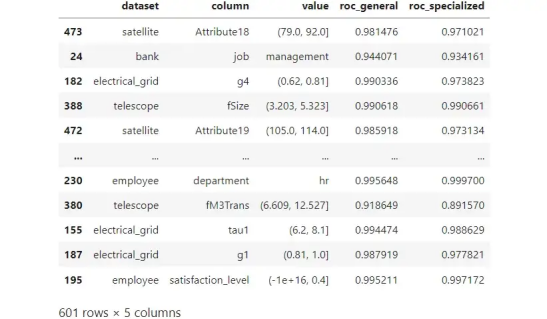12 个真实数据集的模拟结果。每行都是一个段，由数据集、列和值的组合标识。图源作者。601 比较的总结。“general > specialized”表示通用模型的ROC曲线下面积高于专用模型，“specialized > general”则相反。“显着”/“不显着”表明这种差异是否显着。图源作者。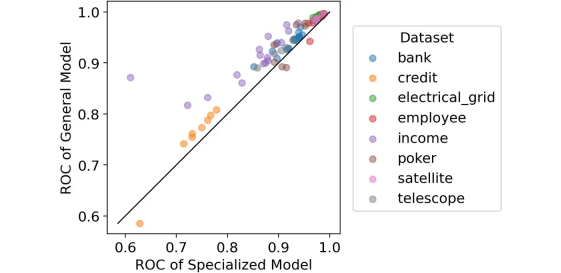# 结论

What Is Better: One General Model or Many Specialized Models?

https://towardsdatascience.com/what-is-better-one-general-model-or-many-specialized-models-9500d9f8751d